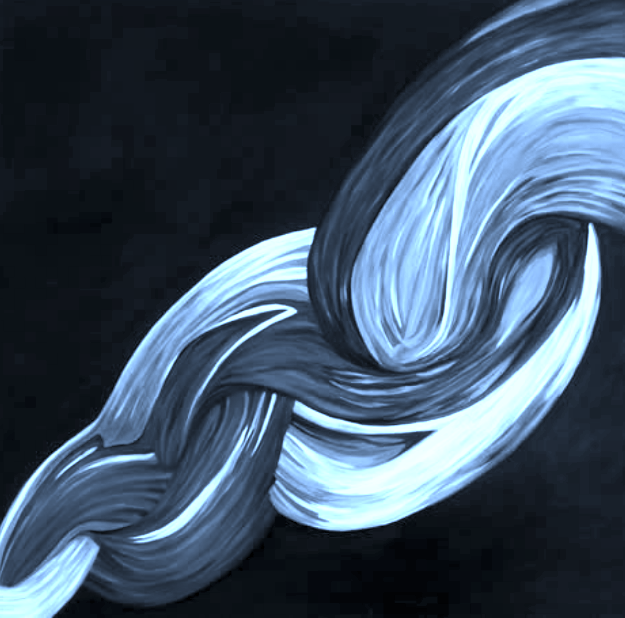# AI forarithmetic curves

AI-assisted maths

AI can predict invariants of low genus arithmetic curves, including those key to the Birch-Swinnerton-Dyer conjecture—a millennium prize problem.

## Machine learning invariants of arithmetic curves

We show that standard machine learning algorithms may be trained to predict certain invariants of low genus arithmetic curves. Using datasets of size around 105, we demonstrate the utility of machine learning in classification problems pertaining to the BSD invariants of an elliptic curve (including its rank and torsion subgroup), and the analogous invariants of a genus 2 curve. Our results show that a trained machine can efficiently classify curves according to these invariants with high accuracies (> 0.97). For problems such as distinguishing between torsion orders, and the recognition of integral points, the accuracies can reach 0.998.